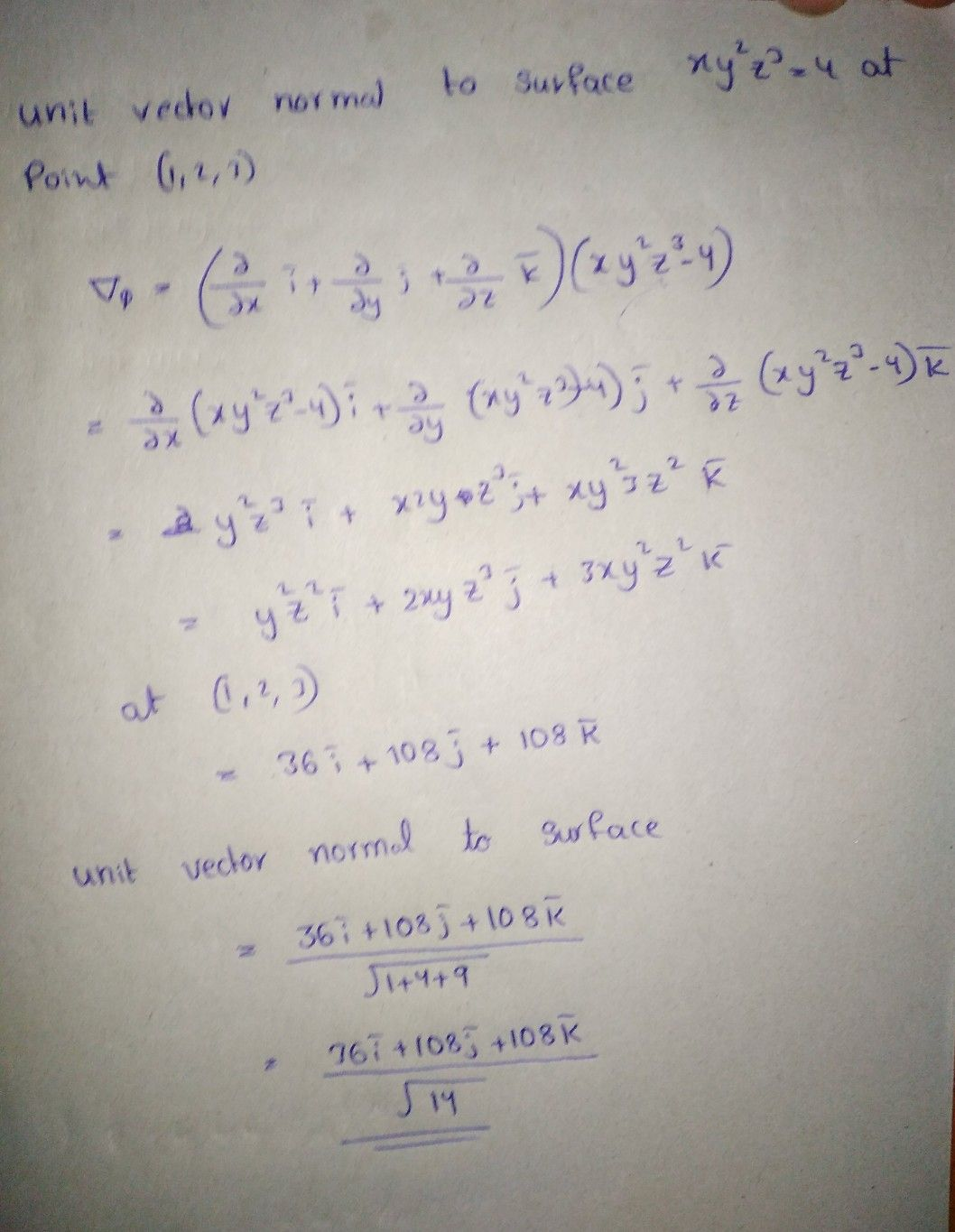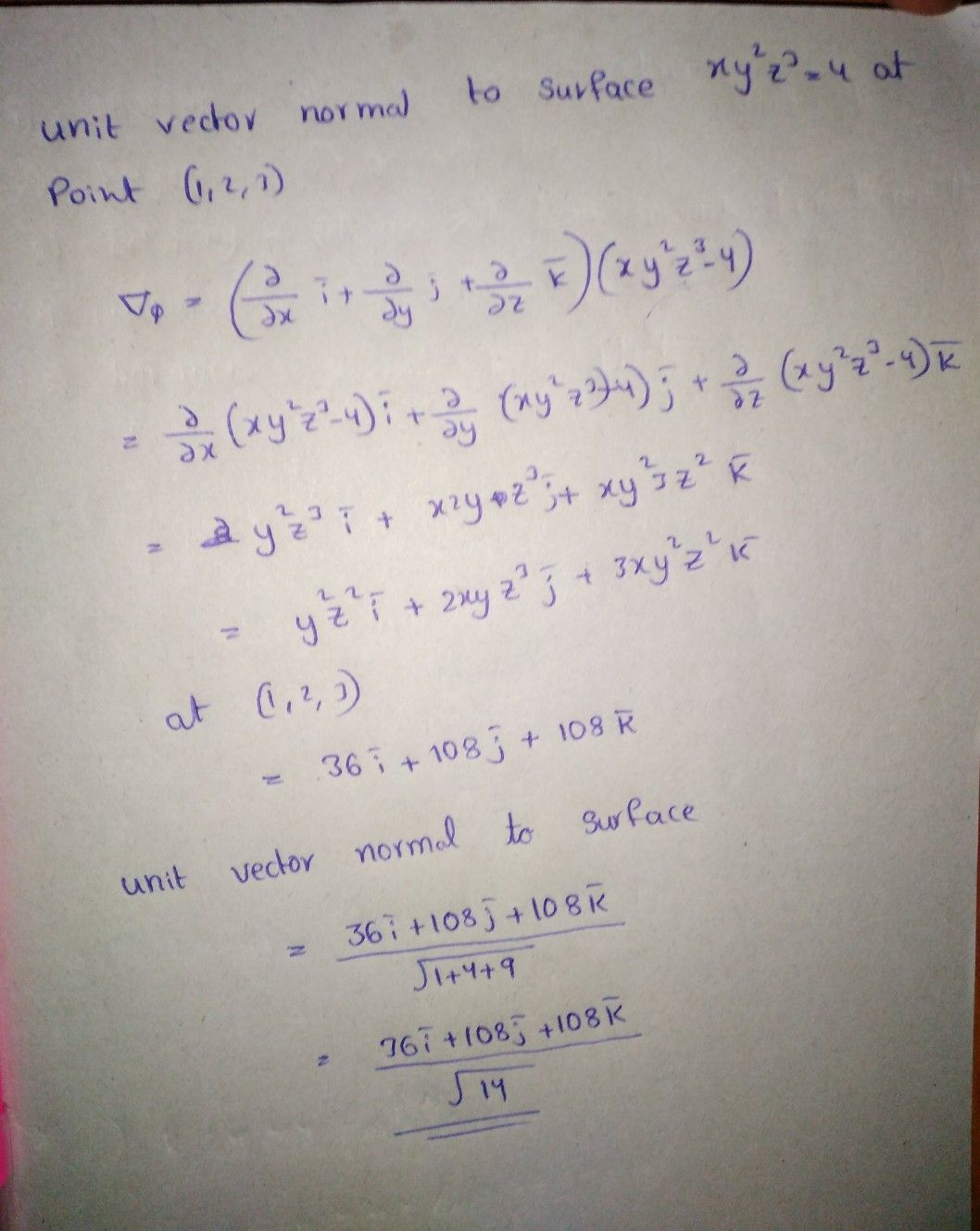Symbol
Problem$7.$ $\left($ (a) $\right)$ Find the unit vector normal to the surface $xy^{2}z^{3}=4at$ the point $\left(1,2,3\right).$
Other
Search count: 106
SolutionQanda teacher - Aravind